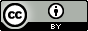MATH 135

Created: 2015-03-29
Status: notes; belief: possible

This is my course review for math 135 at the University of Washington. I took the course in winter 2015 with Ebru Bekyel. This class is a continuation of math 134 (and part of the math 13X sequence at UW). You can look at the course webpage (archived as of the end of the quarter).

The course covered sequences (especially sequences defined recursively by contraction mappings), series (convergence theorems), linear ordinary differential equations (mostly second order, where the series method is I think the only topic not covered in the regular differential equations course, 307), and some vector calculus.

The course material was more challenging for me than in 134, because the latter two parts of the course (differential equations and vector calculus) were new to me. Compared to 134, however, the course was almost entirely computational1.

The test scores did seem a bit “unstable” in the sense that since there were only 16 people taking the course (compared to 23 in math 134, and something like 30 during some years, if you check the timeschedules from years ago), just a few people in the course could really affect the overall score distribution significantly—in fact, for the second midterm, missing even four or five points put you below the median (I forget which). I’m actually not sure how this played a role in the final grades though (I mean, assuming that the 7 worst-scoring students left the course between 134 and 135, obviously if the curve stayed the same then most people would end up getting a worse grade overall).

1. However, the most interesting problem in the whole course, I think, ended up being the following proof problem (from homework 6):

Suppose that $$y_1$$ and $$y_2$$ are a fundamental set of solutions of the ODE $$y'' + p(t)y' + q(t)y =0$$ on the interval $$-\infty < t < \infty$$, where $$p$$ and $$q$$ are continuous for all $$t$$. Prove that there is one and only one zero of $$y_1$$ between two consecutive zeros of $$y_2$$.